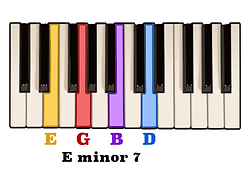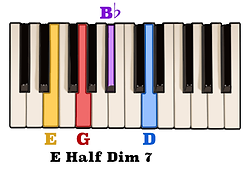top of page

## Seventh Chords Construction | Half Diminished Seventh Chords (4 of 5)

Throughout this video series we have learned how the two components which construct a seventh chord are a triad and an interval of a seventh above its root note. A half diminished seventh chord is called so because only the triad component is diminished, while the interval of a seventh is minor.

Now, the notes of a diminished triad are very similar to the notes of a minor triad with the exception of a flattened fifth.For example, if the notes of an A minor triad are A, C, and E,then the notes of a diminished triad would be A, C, and E-flat.

By adding an interval of a minor seventh above the note A - the note G - to the notes of an A diminished triad we would then be playing an A half diminished seventh chord.Likewise, the notes of a D diminished triad are D, F, and A-flat.Playing these notes together with the note C would create a D half diminished seventh chord.

Another name for a diminished seventh chord is minor seventh flat five. In other words, a half diminished seventh chord may also be looked at as being a minor seventh chord with a flattened fifth.

Here are the notes of a C minor seventh chord.If we simply flatten the note G by one half step to the note G-flat we will have created a C minor seventh flat five chord.In that same way, the notes of an E minor seventh chord are E, G, B, and D.By flattening the note B one half step to the note B-flat we would then be playing the notes of E minor seventh flat five, also known as E half diminished seventh.Another way to construct a half diminished seventh chord above any given note is by following a specific pattern of intervals. The interval pattern which constructs a half diminished seventh chord is minor third, minor third, and Major third.For example, an interval of a minor third above the note B is the note D, another minor third above the note D is the note F, and an interval of a Major third above F is the note A.Here we can see that the notes of a B half diminished seventh chord are B, D, F, and A.Follow this pattern of intervals to create a half diminished seventh chord above any note.

bottom of page